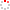CIOMP OpenIR  > 中科院长春光机所知识产出
 Design multiple-layer gradient coils using least-squares finite element method Jia F.; Liu Z.; Zaitsev M.; Hennig J.; Korvink J. G. 2013 Source Publication 1615147XAbstract The design of gradient coils for magnetic resonance imaging is an optimization task in which a specified distribution of the magnetic field inside a region of interest is generated by choosing an optimal distribution of a current density geometrically restricted to specified non-intersecting design surfaces, thereby defining the preferred coil conductor shapes. Instead of boundary integral type methods, which are widely used to design coils, this paper proposes an optimization method for designing multiple layer gradient coils based on a finite element discretization. The topology of the gradient coil is expressed by a scalar stream function. The distribution of the magnetic field inside the computational domain is calculated using the least-squares finite element method. The first-order sensitivity of the objective function is calculated using an adjoint equation method. The numerical operations needed, in order to obtain an effective optimization procedure, are discussed in detail. In order to illustrate the benefit of the proposed optimization method, example gradient coils located on multiple surfaces are computed and characterised. 2013 Springer-Verlag Berlin Heidelberg. Indexed By EI Document Type 期刊论文 Identifier http://ir.ciomp.ac.cn/handle/181722/40604 Collection 中科院长春光机所知识产出 Recommended CitationGB/T 7714 Jia F.,Liu Z.,Zaitsev M.,et al. Design multiple-layer gradient coils using least-squares finite element method[J]. 1615147X,2013. APA Jia F.,Liu Z.,Zaitsev M.,Hennig J.,&Korvink J. G..(2013).Design multiple-layer gradient coils using least-squares finite element method.1615147X. MLA Jia F.,et al."Design multiple-layer gradient coils using least-squares finite element method".1615147X (2013).